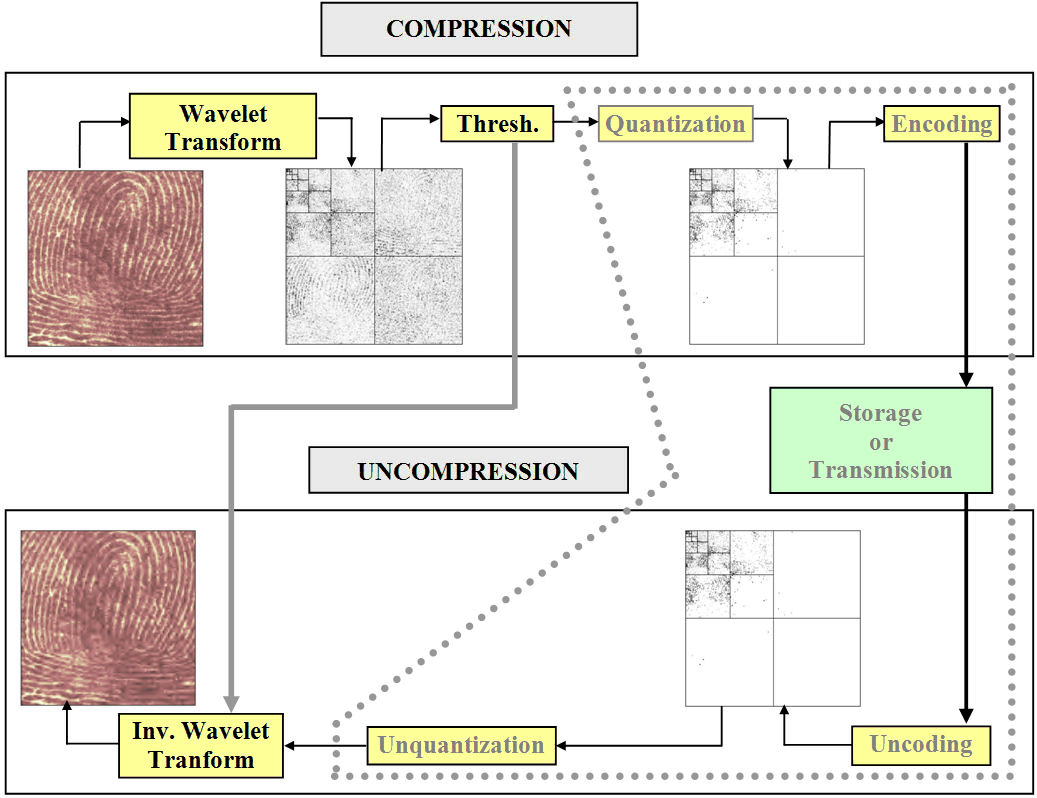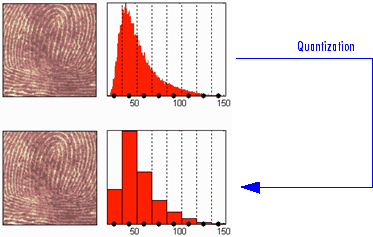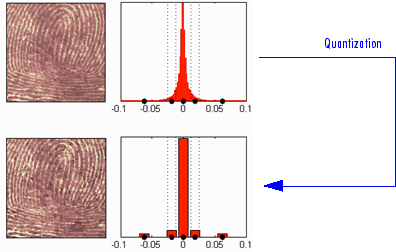## Wavelet Compression for Images

In Wavelet Data Compression, we addressed the aspects specifically related to compression using wavelets. However, in addition to the algorithms related to wavelets like DWT and IDWT, it is necessary to use other ingredients concerning the quantization mode and the coding type in order to deal with true compression.

This more complex process can be represented by the following figure.### Effects of Quantization

Let us show the effects of quantization on the visualization of the fingerprint image. This indexed image corresponds to a matrix of integers ranging between 0 and 255. Through quantization we can decrease the number of colors which is here equal to 256.

The next figure illustrates how to decrease from 256 to 16 colors by working on the values of the original image.We can see on this figure:

• At the top

• On the left: the original image

• On the right: the corresponding histogram of values

• At the bottom

• On the left: the reconstructed image

• On the right: the corresponding histogram of quantized values

This quantization leads to a compression of the image. Indeed, with a fixed length binary code, 8 bits per pixel are needed to code 256 colors and 4 bits per pixel to code 16 colors. We notice that the image obtained after quantization is of good quality. However, within the framework of true compression, quantization is not used on the original image, but on its wavelet decomposition.

Let us decompose the fingerprint image at level 4 with the Haar wavelet. The histogram of wavelet coefficients and the quantized histogram are normalized so that the values vary between –1 and +1. The 15 intervals of quantization do not have the same length.

The next figure illustrates how to decrease information by binning on the wavelet coefficient values of the original image.We can see on this figure:

• At the top

• On left: the original image

• On the right: the corresponding histogram (central part) of coefficient values

• At the bottom

• On the left: the reconstructed image

• On the right: the corresponding histogram (central part) of quantized coefficient values

The key point is that the histogram of the quantized coefficients is massively concentrated in the class centered in 0. Let us note that yet again the image obtained is of good quality.

### True Compression Methods

The basic ideas presented above are used by three methods which cascade in a single step, coefficient thresholding (global or by level), and encoding by quantization. Fixed or Huffman coding can be used for the quantization depending on the method.

The following table summarizes these methods, often called Coefficients Thresholding Methods (CTM), and gives the MATLAB® name used by the true compression tools for each of them.

MATLAB Name

Compression Method Name

`'gbl_mmc_f'`

Global thresholding of coefficients and fixed encoding

`'gbl_mmc_h'`

Global thresholding of coefficients and Huffman encoding

`'lvl_mmc'`

Subband thresholding of coefficients and Huffman encoding

More sophisticated methods are available which combine wavelet decomposition and quantization. This is the basic principle of progressive methods.

On one hand, progressivity makes it possible during decoding to obtain an image whose resolution increases gradually. In addition, it is possible to obtain a set of compression ratios based on the length of the preserved code. This compression usually involves a loss of information, but this kind of algorithm enables also lossless compression.

Such methods are based on three ideas. The two first, already mentioned, are the use of wavelet decomposition to ensure sparsity (a large number of zero coefficients) and classical encoding methods. The third idea, decisive for the use of wavelets in image compression, is to exploit fundamentally the tree structure of the wavelet decomposition. Certain codes developed from 1993 to 2000 use this idea, in particular, the EZW coding algorithm introduced by Shapiro. See [Sha93] in References.

EZW combines stepwise thresholding and progressive quantization, focusing on the more efficient way to encode the image coefficients, in order to minimize the compression ratio. Two variants SPIHT and STW (see the following table) are refined versions of the seminal EZW algorithm.

Following a slightly different objective, WDR (and the refinement ASWDR) focuses on the fact that in general some portions of a given image require more refined coding leading to a better perceptual result even if there is generally a small price to pay in terms of compression ratio.

A complete review of these progressive methods is in the Walker reference [Wal99] in References.

The following table summarizes these methods, often called Progressive Coefficients Significance Methods (PCSM), and gives the MATLAB coded name used by the true compression tools for each of them.

MATLAB Name

Compression Method Name

`'ezw'`

Embedded Zerotree Wavelet

`'spiht'`

Set Partitioning In Hierarchical Trees

`'stw'`

Spatial-orientation Tree Wavelet

`'wdr'`

Wavelet Difference Reduction

`'aswdr'`

`'spiht_3d'`

Set Partitioning In Hierarchical Trees 3D for truecolor images

### Quantitative and Perceptual Quality Measures

The following quantitative measurements and measures of perceptual quality are useful for analyzing wavelet signals and images.

• M S E — Mean square error (MSE) is the squared norm of the difference between the data and the signal or image approximation divided by the number of elements. The MSE is defined by:

$MSE=\frac{1}{mn}\sum _{i=0}^{m=1}\sum _{j=0}^{n=1}{|X\left(i,j\right)-{X}_{c}\left(i,j\right)|}^{2}$

• Max Error — Maximum error is the maximum absolute squared deviation in the signal or image approximation.

• L2-Norm Ratio — L2-norm ratio is the ratio of the squared L2-norm of the signal or image approximation to the input signal or image. For images, the image is reshaped as a column vector before taking the L2-norm

• P S N R — Peak signal-to-noise ratio (PSNR) is a measure of the peak error in decibels. PSNR is meaningful only for data encoded in terms of bits per sample or bits per pixel. The higher the PSNR, the better the quality of the compressed or reconstructed image. Typical values for lossy compression of an image are between 30 and 50 dB. When the PSNR is greater than 40 dB, then the two images are indistinguishable. The PSNR is defined by:

$PSNR=10\cdot {\mathrm{log}}_{10}\left(\frac{{255}^{2}}{MSE}\right)$

• B P P — Bits per pixel ratio (BPP) is the number of bits required to store one pixel of the image. The BPP is the compression ratio multiplied by 8, assuming one byte per pixel (8 bits).

• Comp Ratio — Compression ratio is ratio of the number of elements in the compressed image divided by the number of elements in the original image, expressed as a percentage.

You can find examples illustrating command-line mode and app tools for true compression in Wavelet Compression for Images and the reference page for `wcompress`.